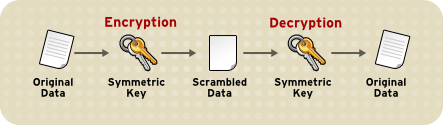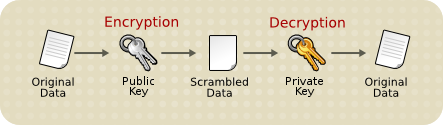# Encryption and Decryption

Encryption is the process of transforming information so it is unintelligible to anyone but the intended recipient. Decryption is the process of transforming encrypted information so that it is intelligible again. A cryptographic algorithm, also called a cipher, is a mathematical function used for encryption or decryption. In most cases, two related functions are employed, one for encryption and the other for decryption.

With most modern cryptography, the ability to keep encrypted information secret is based not on the cryptographic algorithm, which is widely known, but on a number called a key that must be used with the algorithm to produce an encrypted result or to decrypt previously encrypted information. Decryption with the correct key is simple. Decryption without the correct key is very difficult, and in some cases impossible for all practical purposes.

The sections that follow introduce the use of keys for encryption and decryption.

### Symmetric-Key Encryption

With symmetric-key encryption, the encryption key can be calculated from the decryption key and vice versa. With most symmetric algorithms, the same key is used for both encryption and decryption, as shown in Figure 1.Implementations of symmetric-key encryption can be highly efficient, so that users do not experience any significant time delay as a result of the encryption and decryption. Symmetric-key encryption also provides a degree of authentication, since information encrypted with one symmetric key cannot be decrypted with any other symmetric key. Thus, as long as the symmetric key is kept secret by the two parties using it to encrypt communications, each party can be sure that it is communicating with the other as long as the decrypted messages continue to make sense.

Symmetric-key encryption is effective only if the symmetric key is kept secret by the two parties involved. If anyone else discovers the key, it affects both confidentiality and authentication. A person with an unauthorized symmetric key not only can decrypt messages sent with that key, but can encrypt new messages and send them as if they came from one of the two parties who were originally using the key.

Symmetric-key encryption plays an important role in the SSL protocol, which is widely used for authentication, tamper detection, and encryption over TCP/IP networks. SSL also uses techniques of public-key encryption, which is described in the next section.

### Public-Key Encryption

The most commonly used implementations of public-key encryption are based on algorithms patented by RSA Data Security. Therefore, this section describes the RSA approach to public-key encryption.

Public-key encryption (also called asymmetric encryption) involves a pair of keys-a public key and a private key-associated with an entity that needs to authenticate its identity electronically or to sign or encrypt data. Each public key is published, and the corresponding private key is kept secret. Data encrypted with your public key can be decrypted only with your private key. Figure 2 shows a simplified view of the way public-key encryption works.The scheme shown in Figure 2 lets you freely distribute a public key, and only you will be able to read data encrypted using this key. In general, to send encrypted data to someone, you encrypt the data with that person's public key, and the person receiving the encrypted data decrypts it with the corresponding private key.

Compared with symmetric-key encryption, public-key encryption requires more computation and is therefore not always appropriate for large amounts of data. However, it's possible to use public-key encryption to send a symmetric key, which can then be used to encrypt additional data. This is the approach used by the SSL protocol.

As it happens, the reverse of the scheme shown in Figure 2 also works: data encrypted with your private key can be decrypted only with your public key. This would not be a desirable way to encrypt sensitive data, however, because it means that anyone with your public key, which is by definition published, could decrypt the data. Nevertheless, private-key encryption is useful, because it means you can use your private key to sign data with your digital signature-an important requirement for electronic commerce and other commercial applications of cryptography. Client software such as Firefox can then use your public key to confirm that the message was signed with your private key and that it hasn't been tampered with since being signed. "Digital Signatures" describes how this confirmation process works.

### Key Length and Encryption Strength

Breaking an encryption algorithm is basically finding the key to the access the encrypted data in plain text. For symmetric algorithms, breaking the algorithm usually means trying to determine the key used to encrypt the text. For a public key algorithm, breaking the algorithm usually means acquiring the shared secret information between two recipients.

One method of breaking a symmetric algorithm is to simply try every key within the full algorithm until the right key is found. For public key algorithms, since half of the key pair is publicly known, the other half (private key) can be derived using published, though complex, mathematical calculations. Manually finding the key to break an algorithm is called a brute force attack.

Breaking an algorithm introduces the risk of intercepting, or even impersonating and fraudulently verifying, private information.

The key strength of an algorithm is determined by finding the fastest method to break the algorithm and comparing it to a brute force attack.

For symmetric keys, encryption strength is often described in terms of the size or length of the keys used to perform the encryption: in general, longer keys provide stronger encryption. Key length is measured in bits. For example, 128-bit keys for use with the RC4 symmetric-key cipher supported by SSL provide significantly better cryptographic protection than 40-bit keys for use with the same cipher. Roughly speaking, 128-bit RC4 encryption is 3 x 1026 times stronger than 40-bit RC4 encryption. (For more information about RC4 and other ciphers used with SSL, see "Introduction to SSL.") An encryption key is considered full strength if the best known attack to break the key is no faster than a brute force attempt to test every key possibility.

Different ciphers may require different key lengths to achieve the same level of encryption strength. The RSA cipher used for public-key encryption, for example, can use only a subset of all possible values for a key of a given length, due to the nature of the mathematical problem on which it is based. Other ciphers, such as those used for symmetric key encryption, can use all possible values for a key of a given length, rather than a subset of those values.

Because it is relatively trivial to break an RSA key, an RSA public-key encryption cipher must have a very long key, at least 1024 bits, to be considered cryptographically strong. On the other hand, symmetric-key ciphers can achieve approximately the same level of strength with an 80-bit key for most algorithms.